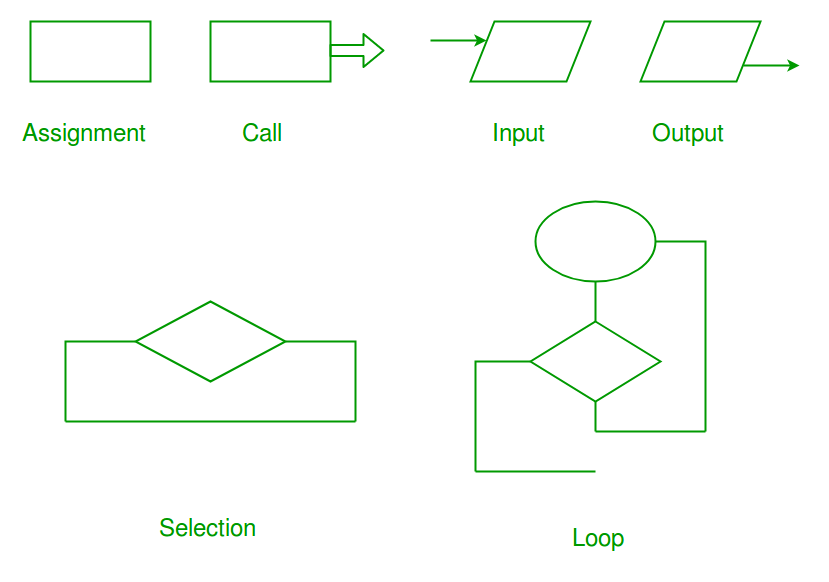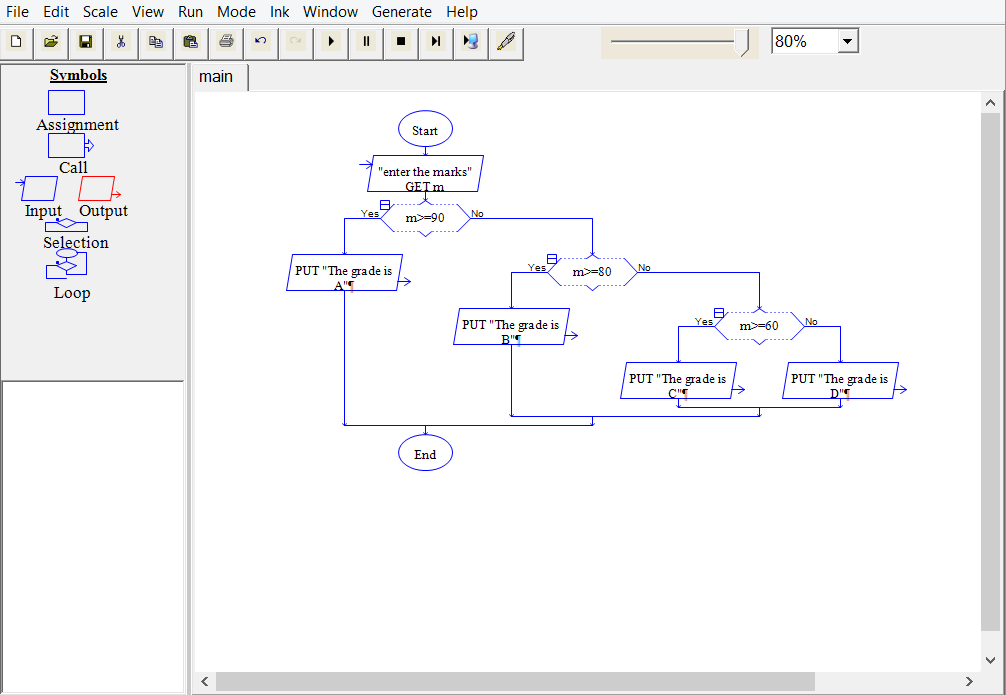Related Articles
RAPTOR Tool – A Flowchart Interpreter
• Difficulty Level : Expert
• Last Updated : 09 Feb, 2018

RAPTOR(Rapid Algorithmic Prototyping Tool for Ordered Reasoning) is a free graphical authoring tool created by Martin C. Carlisle, Terry Wilson, Jeff Humphries and Jason Moore, designed specifically to help students visualize their algorithms and avoid syntactic baggage.
Students can create flow-chart for a particular program and raptor tool will generate code for it in various programming languages, such as C, C++, Java and so on.

Symbols in RAPTOR

Raptor has 6 types of symbols, each of which represents a unique kind of instruction. They are – Assignment, Call, Input, Output, Selection and Loop symbols. The following image shows these symbols-RAPTOR Program Structure

A RAPTOR program consists of connected symbols that represent actions to be executed.

1. The arrows that connect the symbols determine the order in which the actions are performed.
2. The execution of a RAPTOR program begins at the Start symbol and goes along the arrows to execute the program.
3. The program stops executing when the End symbol is reached.With the help of Generate option, the generated C++ code for the above flow chart is:

 `// CPP program for illustrating RAPTOR``#include ``#include `` ` `using` `namespace` `std;``int` `main()``{``   ``string raptor_prompt_variable_zzyz;``   ``float` `m;`` ` `   ``raptor_prompt_variable_zzyz =``"enter the marks"``;``   ``cout << raptor_prompt_variable_zzyz << endl;``   ``cin >> m;``   ``if` `(m>=90)``   ``{``      ``cout << ``"The grade is A"` `<< endl;   }``   ``else``   ``{``      ``if` `(m>=80)``      ``{``         ``cout << ``"The grade is B"` `<< endl;      }``      ``else``      ``{``         ``if` `(m>=60)``         ``{``            ``cout << ``"The grade is C"` `<< endl;         }``         ``else``         ``{``            ``cout << ``"The grade is D"` `<< endl;         }``      ``}``   ``}`` ` `   ``return` `0;``}`

In this way, any algorithm can be visualised by the students, and it can be converted into a code using the raptor tool.

This article is contributed by Mrigendra Singh. If you like GeeksforGeeks and would like to contribute, you can also write an article using contribute.geeksforgeeks.org or mail your article to contribute@geeksforgeeks.org. See your article appearing on the GeeksforGeeks main page and help other Geeks.

Please write comments if you find anything incorrect, or you want to share more information about the topic discussed above.My Personal Notes arrow_drop_up